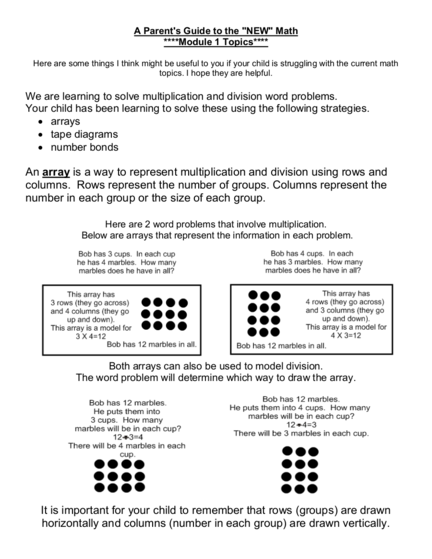# A Parent's Guide to solve Multiplication and Division Word ProblemsContributed by:1. A Parent's Guide to the "NEW" Math
****Module 1 Topics****
Here are some things I think might be useful to you if your child is struggling with the current math
topics. I hope they are helpful.
We are learning to solve multiplication and division word problems.
Your child has been learning to solve these using the following strategies.
 arrays
 tape diagrams
 number bonds
An array is a way to represent multiplication and division using rows and
columns. Rows represent the number of groups. Columns represent the
number in each group or the size of each group.
Here are 2 word problems that involve multiplication.
Below are arrays that represent the information in each problem.
Both arrays can also be used to model division.
The word problem will determine which way to draw the array.
It is important for your child to remember that rows (groups) are drawn
horizontally and columns (number in each group) are drawn vertically.
2. A tape diagram is another way to represent
information in a word problem.
We are learning to use tape diagrams to solve problems that
involve both multiplication and division.
A tape diagram starts with a rectangle. The students must
label the tape diagram using information from the problem.
Since multiplication and division problems always involve
number of groups, size of each group and the total, your
child should label 3 things in the diagram.
Since one of the 3 labeled items will be the unknown fact, we
label it with a question mark. The actual answer to the
problem is revealed in sentence form after the diagram is
completed.
Once the tape diagram is labeled with all the known and
unknown information, the sections (called units) are filled in.
This can be done using dots or by skip counting and writing
numbers.
Below is an example of a multiplication type problem,
a labeled tape diagram
and a sentence written to show the solution.
3. Karen has a 5 boxes of
Karen have in all?
Tape diagrams can also be used to solve problems involving
4. Here is an example:
She packs them into boxes of 7.
How many boxes does she pack?
5. Tape diagrams are being used in class to solve 2 step word
problems like the one below. Because there are 2 steps, your child
will have to show their work for each step. This is done even if your
child can do the math in his/her head!
Karen has 5 boxes of cookies. She has a
3 of Karen's boxes?
6. Sometimes a second tape diagram is used to represent the
second step of a word problem.
bag costs \$7. Karen also buys one bag of chocolate
chips for \$3. How much did Karen spend at the
market?
7. A number bond is another way to represent information in
a word problem.
Joe has 24 Snickers Bars. He puts them into
piles of 4 so he can eat an equal amount of
bars each day till they are all gone. How
many days will it take for Joe to eat his
Snickers Bars?
8. Have you noticed RDWW on your child's math work?
RDWW stands for Read, Draw, Write and Write.
In order to solve a word problem, the children must: